# Minute-hand

How long distance will travel a large (minute) hand on the clock for 48 minutes, if its length is 56 cm?

s =  281.49 cm

### Step-by-step explanation: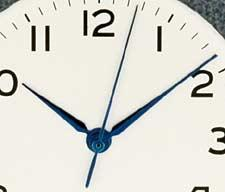Did you find an error or inaccuracy? Feel free to write us. Thank you!Tips to related online calculators
Do you want to convert length units?
Do you want to convert time units like minutes to seconds?

#### You need to know the following knowledge to solve this word math problem:

We encourage you to watch this tutorial video on this math problem:

## Related math problems and questions:

• Minute handWhat is the distance the minute hand of the clock travels in 12 minutes, if the diameter of the clock is 30 cm and the hand extends to a distance of 2 cm from the edge of the clock?
• ClocksHow long track travel the second hand of a clock in 46 hours, which is 4 cm long.
• ClocksHow will long path walk the end of the clock's minute-hand long 7 cm for 330 minutes? How long will walk in the same time hour-hand?
• Clock handsThe second hand has a length of 1.5 cm. How long does the endpoint of this hand travel in one day?
• Clock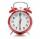What distance will pass end of 8 cm long hour hand for 15 minutes?
• Clocks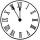What distance will describe the tip of minute hand 6 cm long for 20 minutes when we know the starting position with finally enclose hands each other 120°?
• Time clockWhat are the angle of the hour hand and the minute hand if it is 0:40?
• HandsThe clock shows 12 hours. After how many minutes will angle between the hour and minute hand 90°? Consider the continuous movement of both hands hours.
• LunchLunch is given to seniors from 12:15 to 12:40 during the Coronavirus pandemic. What angle will the minute hand of clock describe during this time?
• Little hand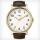What angle shifted little hand on the clock after one hour and 38 minutes?
• The small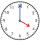The small hand of the wall clock is 15 cm long. What a long journey the end of the hour hand in 15 minutes will describe. (b - in 2 hours)?
• WalkersWalker, which makes 120 steps per minute, make distance from point A to point B for 55 minutes. The length of his step is 75cm. For how long does this distance go boy who will do 110 steps 60 cm long in a minute?
• Central angleWhat is the length of the arc of a circle with a diameter of 46 cm, which belongs to a central angle of 30°?
• ClocksThe length of the minute hand is 12 cm. What track in meters will its end point describe in a week?
• Minute hand v2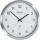In how many minutes describe the minute hand angle 60 degrees?
• Speed of clockIn how many minutes will the hour hand pass a straight angle and a right angle?
• Clock Tower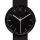What angle is betwenn hands on Clock Tower when show 17 hours and 35 minutes?